# Shortcut keys for inserting symbols and templates into the equation

Word
In Word for Microsoft 365 equations many mathematical symbols can be inserted using the \+name of the symbol (how to insert Greek symbols, see Shortcut keys for inserting Greek symbols into the equation):

### Some of the commonly used symbols: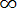\infty - Infinity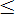\leq - Less than or equal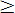\geq - Greater than or equal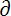\partial - Partial differential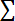\sum - Summa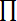\prod - Product Sign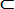\subset - Contained in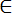\in - Element of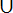\cup - Union (if you want to see big symbol, enter \bigcup)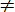\neq - Not equal to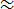\approx - Almost equal to (asymptotic to)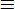\equiv - Identical to (equivalent)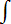\int - Integral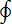\oint - Contour integral

How to use all these symbols outside the equation, select the option Use Math AutoCorrect rules outside of math regions in the Word Options. How to do it, see Choosing Math AutoCorrect Options.

See also this tip in French: Raccourcis clavier pour entrer des symboles et des modèles dans équations.

If you have any questions or suggestions, please feel free to ask OfficeToolTips team.